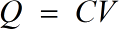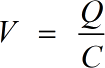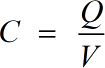Request a Tool

Capacitance Calculator

To calculate q=cv, v=q/c and c=q/v use this Capacitance or potential difference Calculator.

Output

Electrical Charge
0

Formula• Q = Electrical Charge
• V = Potential Difference
• C = Capacitance

Defination / Uses

When a subatomic particle is placed in an electric or magnetic field, it has an electric charge, which causes it to experience a force.

Output

Potential Difference
0

Formula• V = Potential Difference
• C = Capacitance
• Q = Electrical Charge

Defination / Uses

The amount of energy required to move a unit charge from one point to another is defined as the potential difference between any two points.

Capacitance
0

Formula• C = Capacitance
• Q = Electrical Charge
• V = Potential Difference

Defination / Uses

The ability of a capacitor to store energy in an electric field is referred to as capacitance. An electronic component known as a capacitor is used to store this energy. The symbol 'C' is used to represent capacitance. The capacitance C and the voltage differential V applied across the capacitor determine the amount charged. When a constant voltage is applied to the capacitor, it creates a pair of plates.